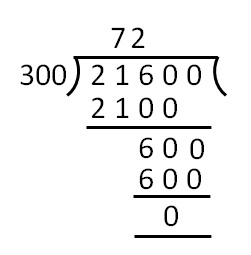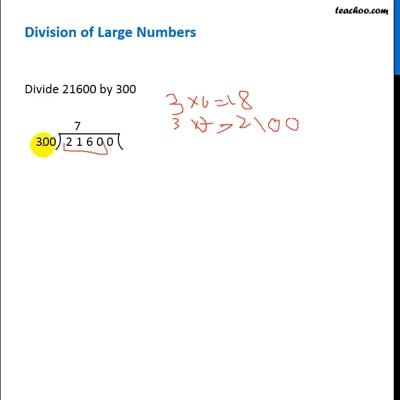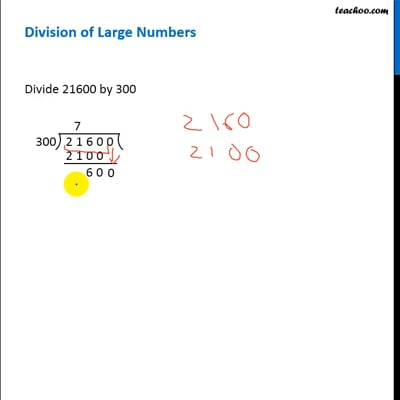Division of large numbers

Chapter 1 Class 6 Knowing our Numbers
Concept wise

## Division of Large Numbers

Divide 21600 by 300So, division is 72This video is only available for Teachoo black usersThis video is only available for Teachoo black users

### Transcript

Division of Large Numbers Suppose we want to divide 21600 by 300 We follow these steps 1. We write the numbers with a division symbol. Putting 21600 inside, 300 on the left side. 2. Now, since the divisor has 300 has 3 digits, we take first 3 digits of 21600 - 216, and see if we can divide it by 300. 3. Since 300 is greater than 216, we take first four digits, 2160. So, we need to divide 2160 with 300 4. Now, we know 3 x 7 = 21, so 300 x 7 = 2100. Writing 2100 below 2160, and 7 on top. 5. Remainder comes to 60. 6. Putting the remaining 0 from top to 60. The number becomes 600. 7. So, we need to divide 600 by 300. 8. Since 300 x 2 = 600, writing 2 on top, next to 7 and 600 below 600. 9. Remainder is 0. So, our division is done 10. The number on top is the division - 72 So, 21600/300 = 72